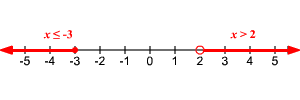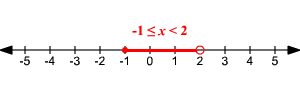# Compound Inequalities

A compound inequality (or combined inequality ) is two or more inequalities joined together with or or and .

To be a solution of an or inequality, a value has to make only one part of the inequality true.  To be a solution of an and inequality, it must make both parts true.

For example:

$x\le -3$ or $x>2$When two inequalities are joined with and , they are often written simply as a double inequality, like:

$-1\le x<2$

(In other words, $x\ge -1$ and $x<2$ .)## Solving Compound Inequalities

Consider the compound inequality $-2 .

To solve it, we need to subtract $3$ -- not "from both sides", as you would do in a normal inequality, but from all THREE parts of the compound inequality.

$\begin{array}{l}-2-3

In case you need to solve an "or" inequality, you can just treat the two inequalities separately.

$-3x+1<-5$ OR $2x+2<-10$

To solve the left part, first subtract 1 from both sides.

$-3x<-6$

Then divide both sides by $-3$ . Remember to reverse the inequality.

$x>2$

For the second part, subtract $2$ from both sides.

$2x<-12$

Then divide both sides by $2$ .

$x<-6$

So, the solution of the compound inequality is

$x<-6$ OR $x>2$